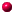For Honours Students Projects and Essays Honours CourseNonlinear Integrable Difference Equations The field of integrable difference equations is only about 15 years old, but has already caused great interest amongst physicists (in the theory of random matrices, string theory, or quantum gravity) and mathematicians (in the theory of orthogonal polynomials and soliton theory). For each integrable differential equation there are, in principle, an infinite number of discrete versions. An essay in this area would provide a critical survey of the many known difference versions of famous equations, such as the Korteweg-de Vries equation and the Painlevé equations, comparisons between them, and analyse differing evidence for their integrability. The field is so new that many calculations remain to be done: including derivations of exact solutions and transformations for discrete integrable equations. Blowing up the solution space of Integrable Systems Imagine a dynamical system with points where the equations become ill-defined, so much so that no solutions can be defined there. What do you do? You blow it up (mathematically)! This technique (known to Newton but not widely used in modern analysis) leads to very detailed information about solutions of non-linear differential equations. In this project, you will learn how to calculate explicit, new information about solutions through such techniques and extend them to discrete equations. For example, there are classes of discrete Painlevé equations for which we know nothing about how their solutions behave as parameters go to special limits. There is a great opportunity in this project to explore such original questions. Integrable Cellular Automata Cellular automata (CA) are discrete systems where the values of solutions (or states)and their independent variables take on only discrete values. A famous example is Conway's Game of Life where each cell only has two states (black or white) and their states evolve according to four very simple rules. The evolution of the states of such CA only depend on the states of their immediate neighbours. It turns out that there are more general CA, called filter parity rules, whose evolution depends on a semi-infinite set of cells at each time step. In this project, you can carry out mathematical analysis of the states of integrable CA. If you are interested in programming, you can also write java applets to simulate the collection of distinct states of such CA. Exponential Asymptotics Near an irregular singular point of a differential equation, the solutions usually have divergent series expansions. Although these can be "summed" in some way to make sense as approximations to the solutions, they do not provide a unique way of identifying a solution. There is a hidden free parameter which has an effect like the butterfly in chaos theory. This problem has been well studied for many classes of nonlinear ODEs but almost nothing is known for PDEs and not much more is known for difference equations. This project would include studies of a model PDE, like the famous Korteweg-de Vries equation near infinity, or a difference equation like the string equation that arises in 2D quantum gravity. Mathematical Immunology After almost three decades of knowledge about HIV/AIDS, it was only recently discovered that a cellular automata model is capable of replicating the long three-phase cycle in clinical data on T-cell populations. However, agreement with reality is still lacking. Asymptotics of cellular automata appear to be crucial to understanding this cycle. However, currently no such theory is known. This project can go in many directions. One direction is to consider different cellular-automata models for disease transmission. A second direction is to develop mathematical methods to work out limiting behaviours of cellular automata. Integrable Systems: Integrable systems is a subject that is full of paradoxes and miraculous resolutions. They were discovered half a century ago, through a paradox uncovered by Fermi, Pasta and Ulam, who studied heat distribution in metals through a lattice model. They expected that an initial distribution of heat in such a model would die away with time, much like a metallic bar cools down over time. But to their surprise, they found recurrences of initial heat distributions. This paradox engaged many famous scientists of that time and was finally resolved by Kruskal and Zabusky who discovered astonishingly well-behaved solutions that they called "solitons" in the continuum limit of the lattice model. Their discoveries led to a completely new field: completely integrable systems, which is now regarded as one of the most profound advances of twentieth century mathematics. In this course, I will present this theory and its modern development for discrete non-linear systems.Home PagePostgraduate StudentsRecent ResearchPapers Last modified: 21 September 2011 by N.Joshi